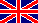# Unit conversionOn this page you can find some details about methods of unit conversion, equations, and you can do also some unit conversions. VLE - Calc has an easy-to-use converter tool to display numeric data in several units.

The formula in the demo tables refreshes automatically depending on the selected units.

## Conversion of temperature

Enter the temperature value you wish to convert into the cell of original value and select your units by clicking on the blue unit labels.

 Original Converted Formula value unit value unit K 20.00 °C [°C] = [K] - 273.16

## Conversion of pressure

Enter the pressure value you wish to convert into the cell of original value and select your units by clicking on the blue unit labels.

 Original Converted Formula value unit value unit bar 100 kPa [kPa] = [bar] * 100

## Conversion of concentration

Calculation of different concentration types needs a little bit more effort than other simple conversions, because some physical data of all of the components have to be given. Enter the concentration values as well as molecular weight and liquid density data into the corresponding cells and select your units by clicking on the blue unit labels.

Note: the temperature-dependency of density and the contraction of volume in mixtures are neglected by these calculation methods.

concentration / composition converter demo (up to 3 components)
Component data Original
concentration
Converted
concentration
Formula
Mol. weight
[M]
Density
[D]
g/mol g/cm3 mol/mol mass% ---
100
[mass%]i = 100% *  [mol/mol]i*Mi
 N [mol/mol]j*Mjj=1
0
0

## Conversion of specific heat and heat of vaporization

Enter the value you wish to convert into the cell of original value and select your units by clicking on the blue unit labels. For molar to mass and vice versa conversions you have to give molar mass too.

 Mol. weight [M] Propertyto convert Original Converted Formulas value unit value unit value unit g/mol specific heat kJ/kg*K 0 J/mol*K [J/mol*K] = [kJ/kg*K] * M g/mol heat of vaporization kJ\mol 0 kJ\kg [kJ/mol] = [kJ/kg]*1000 / M

Lap teteje        KezdõoldalAz Interneten
ezen az oldalon

Useful tools

solubility.info
Predict solubility in water and organic solvents

process-flow-diagram.com
Calculate material flow and draw flowsheet of your chemical processes in a minute.